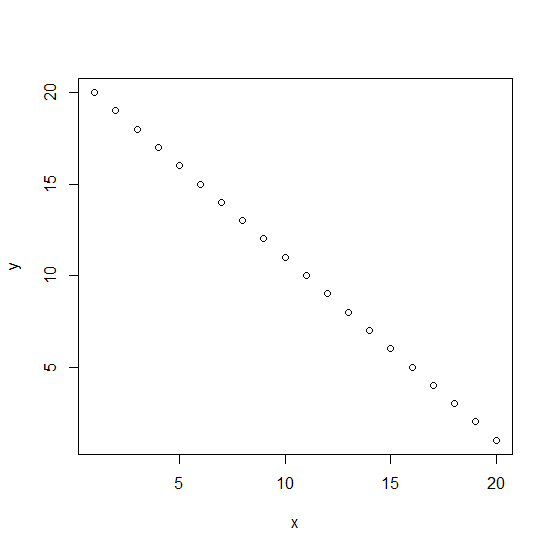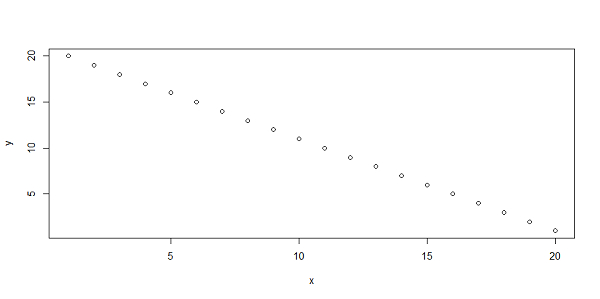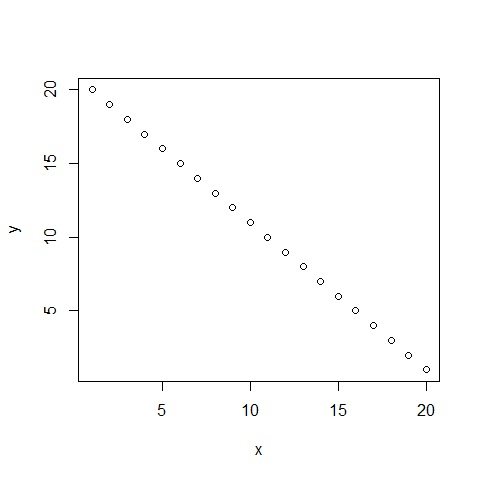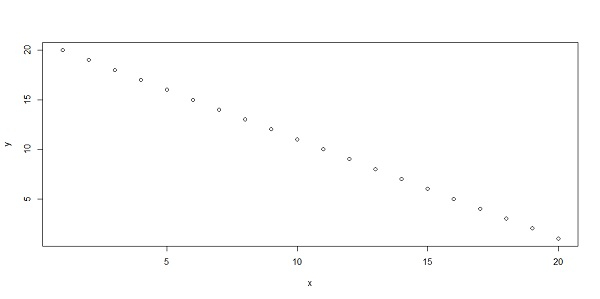# How to create a plot in R with a different plot window size using plot function?

R ProgrammingServer Side ProgrammingProgramming

We can create plots in R with having different plot window sizes. This will be helpful when we want to express X-axis or Y-axis differently. Also, the change in the size of the plot window will help us to paste the plot in places that are short or large. For example, if we want to present the plot in a business meeting then we can increase its size and if we want to publish it in a paper then its size can be decreased.

## Example

Consider the below vectors x and y −

> x<-1:20
> y<-20:1

Plotting with default −

> plot(x,y)

## OutputPlotting with 10-inch-wide and 5 inch in height −

> dev.new(width=10, height=5, unit="in")
> plot(x,y)

## OutputPlotting with 10-cm-wide and 5-cm in height −

> dev.new(width=5, height=5, unit="cm")
> plot(x,y)

## OutputPlotting with 100x50 pixel −

> dev.new(width=100, height=50, unit="px")
> plot(x,y)

## Output